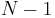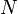# Pointer

This article is intended to provide an introduction to and critical analysis of the concept of a pointer. Despite the samples of code shown below for various languages, it is not intended to provide a reference on how to use pointers in specific languages.

A pointer is an object that contains information about the address of another object in memory (called the referent or the pointee) and which may be used to access that object. Accessing an object using a pointer to it rather than by referring to the object itself is known as indirection; using a pointer is said to add a level of indirection. When we use a particular pointer to access its referent, we say that we are dereferencing the pointer.

## Definition

The usage of the term pointer among programmers does not quite mirror the general definition given above. Whether or not certain objects can be considered pointers is subject to dispute. We consider a few examples:

### C

In C, a pointer is a first class object. A pointer may be used in any place where it is appropriate. Pointers may be assigned to each passed to functions, and returned from functions. They may be converted to other values, they may be printed on the screen, and they may be read from and written to files. To every C data type `T` there corresponds a pointer type `T*`, and this is true even of pointers themselves; a pointer may point to another pointer. The programming community is in consensus that these objects are considered true pointers.

The standard does not prescribe the nature of a pointer; however, C is, by design, close to the machine and efficient with computing time and memory, and, therefore, in essentially all implementations of C, a pointer is a raw memory address, that is, one that contains no more and no less information than is required in order to unambiguously specify the address of an object in primary storage (RAM/ROM) or virtual memory in a form that the CPU can use directly. Today, the vast majority of computing devices in existence operate in flat memory addressing; here, a pointer is simply an unsigned integer from 0 to$N-1$ (where$N$ is the total size of available memory in bytes). The pointer that compares equal to the integer 0 is called the null pointer and is taken to mean a pointer that does not point to anything.

Pascal and its descendants have pointers that are similar to C pointers, but more limited (in that they cannot be operands to arithmetic operators, nor can they be read and written like numeric and character types).

#### Examples

```int x = 123;
int* p = &x;
*p = 456;
printf("%d\n", x); /* This prints "456" */```

Note that `x` is an integer and `p` is a pointer to integer. In line 2, `p` is made to point to `x`. Beyond this point, `*p` and `x` are semantically indistinguishable; using the asterisk operator on `p` is said to dereference the pointer, providing access to the referent. This remains the case until either `x` or `p` goes out of scope, or `p` is reassigned to point somewhere else.

```#include <stdio.h>
void foo(int z)
{
z = 456;
}
void bar(int* p)
{
*p = 456;
}
int main(int argc, char** argv)
{
int x = 123;
foo(x);
printf("%d\n", x); /* This prints "123" */
bar(&x);
printf("%d\n", x); /* This prints "456" */
return 0;
}```

This example shows how pointers may be used to pass by reference. If an integer variable is passed into a function, then a copy of the variable is made; any changes made to the parameter within the function will leave the variable in the caller unaffected. However, passing a pointer to `x` rather than `x` itself merely copies the address information of `x`, which, within the body of `f`, may still be used to refer to `x` itself.

#### Function pointers

Pointers to functions are also allowed. Unlike pointers to data types, these point to code, not data.

```#include <stdio.h>
void f(int x)
{
printf("%d\n", x);
}
int main()
{
void (*p)(int) = &f;
(*p)(123); // prints "123"
return 0;
}```

The type `void (*)(int)` is a pointer to function of `int` returning `void`. When `p` is declared, it is made to point to the function `f`. Then, `*p` will refer to `f`. A function pointer is also a fully first-class data type. Pointers to function pointers are allowed, as are pointers to functions taking or returning function pointers; however, the syntax quickly becomes confusing.

(Note that for function pointers the use of `&` and `*` are optional; the syntax `void (*p)(int) = f;` is correct, as is `p(123);`)